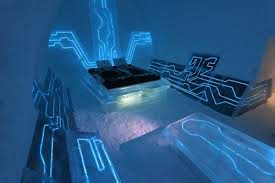Published on Sep 16, 2019

### Abstract

Energy Meter Rate Display project is microcontroller based Energy Monitor and calculating the fare, and using the prepaid card for paying the amount to an EB substation. This project will be very much useful to EB distribution system. This project will not only useful to monitor and transfer energy, and also it can show the electricity theft.

If suppose we implement the project for a complete network in an area, that will be consuming very huge amount and it can calculate the energy transmission and energy consumption. By using the above two values we can calculate the transmission loses we are going to implement an energy meter with digital transmission for single house.

In a house to calculate the energy we have to monitor the existing energy meter reading. The energy meter readings are taken by an sensor, which is kept in between the slotted disk of the analog energy meter. Then the number of pulses will be counted.

Then the microcontroller will display the energy output in seven segment LED display. The accuracy of the meter depends on the existing meter. The sim card is actually a memory card which is used to detect the amount from the card